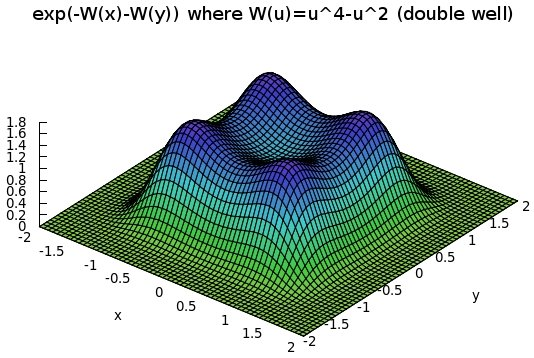Let ${V:\mathbb{R}^d\rightarrow\mathbb{R}}$ be a smooth “potential”. We do not assume that ${e^{-V}}$ is Lebesgue integrable for the moment. Let ${\mu}$ be the positive Borel measure on ${\mathbb{R}^d}$ with Lebesgue density function ${e^{-V}}$. Let us assume additionally that one the following properties holds:

1. ${\lim_{\left|x\right|\rightarrow\infty}V(x)=\infty}$ and ${\inf_{\mathbb{R}^d}(\left|\nabla V\right|^2-\Delta V)>-\infty}$
2. there exists ${a,b\in\mathbb{R}}$ such that ${x\cdot \nabla V(x) \geq -a\left|x\right|^2-b}$ for all ${x\in\mathbb{R}^d}$.

These conditions ensure the non explosion of the Langevin-Kolmogorov Markov diffusion process ${(X_t)_{t\geq0}}$ on ${\mathbb{R}^d}$ solving the stochastic differential equation, driven by a standard Brownian Motion,

$\begin{cases} dX_t&=\sqrt{2}\,dB_t-\nabla V(X_t)\,dt\\ X_0&=x \end{cases}$

see e.g. the book of Royer. It can be shown without difficulty that these conditions on the potential are satisfied if for instance there exists a constant ${\kappa\in\mathbb{R}}$ such that ${\nabla^2 V(x) \succcurlyeq \kappa I_d}$ for every ${x\in\mathbb{R}^d}$. Here “${\succcurlyeq}$” stands for the Loewner partial order on quadratic forms (Hermitian matrices). If ${\kappa>0}$ then ${e^{-V}}$ is uniformly log-concave and is Lebesgue integrable. By the way, a nice result due to Caffarelli and based on the Brenier theorem and the Monge-Ampère equation states that ${\mu}$ is then the image of the standard Gaussian law by a ${\kappa}$ Lipschitz map. However, ${e^{-V}}$ can be Lebesgue integrable beyond this log-concavity sufficient condition (e.g. multiple wells potentials). The infinitesimal generator of ${(X_t)_{t\geq0}}$ is the second order differential operator

$L:=\Delta-\nabla V\cdot\nabla.$

The Markov semigroup ${(P_t)_{t\geq0}=(e^{tL})_{t\geq0}}$ is given by ${P_t(f):=\mathbb{E}(f(X_t)|X_0=\cdot)}$. Following e.g. the Definition 2.4.2 in the ABC book, we assume that there exists a nice algebra of functions ${\mathcal{A}}$ for our computations. We define the functional quadratic forms ${\Gamma}$ and ${\Gamma_{\!\!2}}$ by

$2\Gamma(f,g):=L(fg)-fLg-gLf$

and

$2\Gamma_{\!\!2}(f,g):=L\Gamma(f,g)-\Gamma(f,Lg)-\Gamma(g,Lf)$

for every ${f,g\in\mathcal{A}}$. Some algebraic computations reveal that

$\Gamma(f,f)=\left|\nabla f\right|^2 \quad\text{and}\quad \Gamma_{\!\!2}(f,f)=\Vert\nabla^2f\Vert_{HS}^2+\nabla f\cdot\nabla^2V\nabla f. \ \ \ \ \ (1)$

Here ${\left\Vert A\right\Vert_{HS}^2:=\mathrm{Tr}(AA^*)=\sum_{i,j=1}^d|A_{i,j}|^2}$ is the Hilbert-Schmidt norm of the ${d\times d}$ matrix ${A}$. We have the integration by parts formula (${\mu}$ is symmetric invariant for ${L}$)

$\mathcal{E}(f,g):=\int\!\Gamma(f,g)\,d\mu=-\int\!fLg\,d\mu=-\int\!gLf\,d\mu$

for all ${f,g\in\mathcal{A}}$. The functional quadratic form ${\mathcal{E}}$ is the Dirichlet form. We have, using the definition of ${\Gamma_{\!\!2}}$ and the integration by parts formula, for every ${f\in\mathcal{A}}$,

$\int\!\Gamma_{\!\!2}(f,f)\,d\mu = -\int\!\Gamma(f,Lf)\,d\mu = \int\!(Lf)^2\,d\mu\geq0. \ \ \ \ \ (2)$

By combining (2) and (1), we obtain

$\int\!\Vert\nabla^2f\Vert_{HS}^2\,d\mu +\int\!\nabla f\cdot\nabla^2V\nabla f\,d\mu \geq 0. \ \ \ \ \ (3)$

All this is well known, see e.g. the fifth chapter of the ABC book. From now on, we assume that ${\mu(\mathbb{R}^d)<\infty}$. By adding a constant to ${V}$, we may further assume that ${\mu}$ is a probability measure. Since ${\mu}$ is tight, the approximation of linear functions by elements of ${\mathcal{A}}$ in (3) gives

$\int\!\nabla^2 V\,d\mu \succcurlyeq 0 \quad\text{i.e.}\quad \min\mathrm{spec}\left(\int\!\nabla^2V\,d\mu\right)\geq0.$

If we set ${\underline{\lambda}(x):=\min\mathrm{spec}(\nabla^2 V(x))}$ then ${\displaystyle{\int\!\underline{\lambda}\,d\mu\geq0}}$ when ${d=1}$. We may ask:

Question 1 Do we have ${\int\!\underline{\lambda}\,d\mu\geq0}$ if ${d>1}$?

Example 1 (Gaussian and log-concave cases) If ${V(x)}$ is a positive semidefinite quadratic form then ${\mu}$ is up to normalization a Gaussian law, ${\nabla^2V\equiv\rho I_d}$ with ${\rho>0}$ (we assumed that ${\mu}$ is a finite measure), and ${\underline{\lambda}\equiv\rho}$. The answer to Question 1 is thus positive here. More generally, the answer is obviously positive if ${V}$ is more convex than the Gaussian, i.e. ${\nabla^2 V\succcurlyeq \rho I_d}$ on ${\mathbb{R}^d}$.

Example 2 (Gradient type ground state) For every ${x\in\mathbb{R}^d}$, let us pick an eigenvector ${u(x)}$ associated to the eigenvalue ${\underline{\lambda}(x)}$, namely

$u(x)\in\arg\min_{\left\Vert v\right\Vert_2=1}(v\cdot(\nabla^2 V(x))v).$

If ${\mathrm{curl}(u)=0}$ on ${\mathbb{R}^d}$ i.e. ${u=\nabla f}$ on ${\mathbb{R}^d}$ for some ${f}$ (Poincaré lemma for differential forms on contractible manifolds), then, from (3),

$\int\!\underline{\lambda}\,d\mu \geq -n\int\!\left\Vert\nabla u\right\Vert_2^2\,d\mu.$

One may ask if the right hand side may be zero for a non quadratic potential ${V}$. By the way, one can ask under which condition a given field of symmetric matrices

$S:x\in\mathbb{R}^d\mapsto S(x)\in\mathcal{S}_d(\mathbb{R})$

is of the form ${\nabla^2 V=S}$ for some potential ${V}$. The answer is given by the Saint-Venant compatibility conditions, which constitute a matrix version of the Poincaré lemma.

Example 3 (Radial potentials) Suppose that the potential is radial, i.e. takes the form ${V(x)=\varphi(|x|^2)}$ where ${\varphi:\mathbb{R}_+\rightarrow\mathbb{R}}$ is a smooth function. We have

$\nabla V(x)=2\varphi'(|x|^2)x \quad\text{and}\quad \nabla^2 V(x)=2\varphi'(|x|^2)I_d+4\varphi”(|x|^2)xx^\top.$

Consequently, if ${\varphi}$ is convex then ${\underline{\lambda}(x)=2\varphi'(|x|^2)}$, and thus, for ${d\geq2}$,

$\omega_d^{-1}\!\int\!\underline{\lambda}\,d\mu =2\int_0^\infty\!\varphi'(r^2)e^{-\varphi(r^2)}\,r^{d-1}dr =\int_0^\infty\!(-e^{-\varphi(r^2)})’\,r^{d-2}dr$

and therefore

$\omega_d^{-1}\!\int\!\underline{\lambda}\,d\mu =\left[-r^{d-2}e^{-\varphi(r^2)}\right]_0^\infty +(d-2)\int_0^\infty\!e^{-\varphi(r^2)}\,r^{d-3}dr\geq0.$

The answer to Question 1 is thus positive in this case. The answer is probably negative in general when ${\varphi}$ is not convex.

Example 4 (Tensor potentials) Consider the case where ${\mu}$ is a tensor product i.e. ${V(x)=W_1(x_1)+\cdots+W_d(x_d)}$ where all ${W_i:\mathbb{R}\rightarrow\mathbb{R}}$ are smooth. We have

$\nabla V(x)=(W’_1(x_1),\ldots,W’_d(x_d))^\top \quad\text{and}\quad \nabla^2V(x)=\mathrm{Diag}(W_1”(x_1),\ldots,W_d”(x_d)).$

Therefore, ${\underline{\lambda}(x)=\min_{1\leq i\leq d}W_i”(x)}$, and consequently

$\int\!\underline{\lambda}\,d\mu =\int\!\min_{1\leq i\leq d}W”_i(x_i)e^{-W_1(x_1)-\cdots-W_n(x_d)}\,dx.$

If we assume that ${W_1=\cdots=W_d=W}$ then we have the symmetric integral

$\int\!\underline{\lambda}\,d\mu =\int\!\min_{1\leq i\leq d}W”(x_i)e^{-W(x_1)-\cdots-W(x_d)}\,dx_1\cdots dx_d. \ \ \ \ \ (4)$

Let us further specialize to ${d=2}$ and ${W(u)=\alpha u^4- u^2}$ with ${\alpha>0}$ (double well):

$\int\!\underline{\lambda}\,d\mu =2\int_{\mathbb{R}^2}\!(6\alpha\min(x^2,y^2)-1) e^{-\alpha (x^4+y^4)+x^2+y^2}\,dxdy.$

Now a numerical quadrature using the int2d function of the Scilab 5.2.1 software package suggests that this integral is negative for e.g. ${\alpha=1/4}$. The answer to Question 1 seems to be negative in this case.

Note: this post is motivated by a question asked by Raphaël Roux. The answer to Question 1 seems to be sometimes positive, and negative in general. One may then ask for more sufficient conditions on ${V}$ ensuring a positive answer. More recently, Roux came with an interesting further remark: the right hand side of (4) writes ${\mathbb{E}(\min_{1\leq i\leq d}W”(X_i))}$ where ${X_1,\ldots,X_d}$ are i.i.d. of density ${e^{-W}}$, and therefore, if ${W”}$ is bounded with a negative minimum, then ${\min_{1\leq i\leq d}W”(X_i)}$ converges in probability to a negative quantity as ${d}$ goes to infinity.

Last Updated on

This site uses Akismet to reduce spam. Learn how your comment data is processed.

Syntax · Style · .